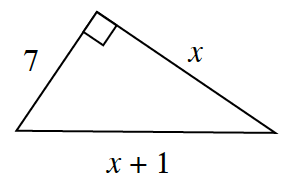### Home > INT2 > Chapter 8 > Lesson 8.1.1 > Problem8-11

8-11.As Randi starts to solve for $x$ in the diagram at right, she writes the equation $7^2 + x^2 = (x + 1)^2$.

1. Is Randi’s equation valid? Explain your thinking.

Notice the right angle.

Yes, she used the Pythagorean Theorem.

2. What is the perimeter of Randi’s triangle?

Once you solve the equation you will need to use the value of $x$ to determine the length of each side of the triangle.